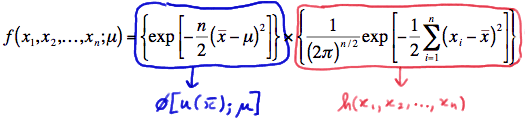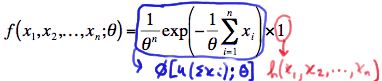# 24.2 - Factorization Theorem

24.2 - Factorization Theorem

While the definition of sufficiency provided on the previous page may make sense intuitively, it is not always all that easy to find the conditional distribution of $$X_1, X_2, \ldots, X_n$$ given $$Y$$. Not to mention that we'd have to find the conditional distribution of $$X_1, X_2, \ldots, X_n$$ given $$Y$$ for every $$Y$$ that we'd want to consider a possible sufficient statistic! Therefore, using the formal definition of sufficiency as a way of identifying a sufficient statistic for a parameter $$\theta$$ can often be a daunting road to follow. Thankfully, a theorem often referred to as the Factorization Theorem provides an easier alternative! We state it here without proof.

Factorization

Let $$X_1, X_2, \ldots, X_n$$ denote random variables with joint probability density function or joint probability mass function $$f(x_1, x_2, \ldots, x_n; \theta)$$, which depends on the parameter $$\theta$$. Then, the statistic $$Y = u(X_1, X_2, ... , X_n)$$ is sufficient for $$\theta$$ if and only if the p.d.f (or p.m.f.) can be factored into two components, that is:

$$f(x_1, x_2, ... , x_n;\theta) = \phi [ u(x_1, x_2, ... , x_n);\theta ] h(x_1, x_2, ... , x_n)$$

where:

• $$\phi$$ is a function that depends on the data $$x_1, x_2, \ldots, x_n$$ only through the function $$u(x_1, x_2, \ldots, x_n)$$, and
• the function $$h((x_1, x_2, \ldots, x_n)$$ does not depend on the parameter $$\theta$$

Let's put the theorem to work on a few examples!

## Example 24-2Let $$X_1, X_2, \ldots, X_n$$ denote a random sample from a Poisson distribution with parameter $$\lambda>0$$. Find a sufficient statistic for the parameter $$\lambda$$.

Because $$X_1, X_2, \ldots, X_n$$ is a random sample, the joint probability mass function of $$X_1, X_2, \ldots, X_n$$ is, by independence:

$$f(x_1, x_2, ... , x_n;\lambda) = f(x_1;\lambda) \times f(x_2;\lambda) \times ... \times f(x_n;\lambda)$$

Inserting what we know to be the probability mass function of a Poisson random variable with parameter $$\lambda$$, the joint p.m.f. is therefore:

$$f(x_1, x_2, ... , x_n;\lambda) = \dfrac{e^{-\lambda}\lambda^{x_1}}{x_1!} \times\dfrac{e^{-\lambda}\lambda^{x_2}}{x_2!} \times ... \times \dfrac{e^{-\lambda}\lambda^{x_n}}{x_n!}$$

Now, simplifying, by adding up all $$n$$ of the $$\lambda$$s in the exponents, as well as all $$n$$ of the $$x_i$$'s in the exponents, we get:

$$f(x_1, x_2, ... , x_n;\lambda) = \left(e^{-n\lambda}\lambda^{\Sigma x_i} \right) \times \left( \dfrac{1}{x_1! x_2! ... x_n!} \right)$$

Hey, look at that! We just factored the joint p.m.f. into two functions, one ($$\phi$$) being only a function of the statistic $$Y=\sum_{i=1}^{n}X_i$$ and the other (h) not depending on the parameter $$\lambda$$:Therefore, the Factorization Theorem tells us that $$Y=\sum_{i=1}^{n}X_i$$ is a sufficient statistic for $$\lambda$$. But, wait a second! We can also write the joint p.m.f. as:

$$f(x_1, x_2, ... , x_n;\lambda) = \left(e^{-n\lambda}\lambda^{n\bar{x}} \right) \times \left( \dfrac{1}{x_1! x_2! ... x_n!} \right)$$

Therefore, the Factorization Theorem tells us that $$Y = \bar{X}$$ is also a sufficient statistic for $$\lambda$$!

If you think about it, it makes sense that $$Y = \bar{X}$$ and $$Y=\sum_{i=1}^{n}X_i$$ are both sufficient statistics, because if we know $$Y = \bar{X}$$, we can easily find $$Y=\sum_{i=1}^{n}X_i$$. And, if we know $$Y=\sum_{i=1}^{n}X_i$$, we can easily find $$Y = \bar{X}$$.

The previous example suggests that there can be more than one sufficient statistic for a parameter $$\theta$$. In general, if $$Y$$ is a sufficient statistic for a parameter $$\theta$$, then every one-to-one function of $$Y$$ not involving $$\theta$$ is also a sufficient statistic for $$\theta$$. Let's take a look at another example.

## Example 24-3Let $$X_1, X_2, \ldots, X_n$$ be a random sample from a normal distribution with mean $$\mu$$ and variance 1. Find a sufficient statistic for the parameter $$\mu$$.

Because $$X_1, X_2, \ldots, X_n$$ is a random sample, the joint probability density function of $$X_1, X_2, \ldots, X_n$$ is, by independence:

$$f(x_1, x_2, ... , x_n;\mu) = f(x_1;\mu) \times f(x_2;\mu) \times ... \times f(x_n;\mu)$$

Inserting what we know to be the probability density function of a normal random variable with mean $$\mu$$ and variance 1, the joint p.d.f. is:

$$f(x_1, x_2, ... , x_n;\mu) = \dfrac{1}{(2\pi)^{1/2}} exp \left[ -\dfrac{1}{2}(x_1 - \mu)^2 \right] \times \dfrac{1}{(2\pi)^{1/2}} exp \left[ -\dfrac{1}{2}(x_2 - \mu)^2 \right] \times ... \times \dfrac{1}{(2\pi)^{1/2}} exp \left[ -\dfrac{1}{2}(x_n - \mu)^2 \right]$$

Collecting like terms, we get:

$$f(x_1, x_2, ... , x_n;\mu) = \dfrac{1}{(2\pi)^{n/2}} exp \left[ -\dfrac{1}{2}\sum_{i=1}^{n}(x_i - \mu)^2 \right]$$

A trick to making the factoring of the joint p.d.f. an easier task is to add 0 to the quantity in parentheses in the summation. That is:Now, squaring the quantity in parentheses, we get:

$$f(x_1, x_2, ... , x_n;\mu) = \dfrac{1}{(2\pi)^{n/2}} exp \left[ -\dfrac{1}{2}\sum_{i=1}^{n}\left[ (x_i - \bar{x})^2 +2(x_i - \bar{x}) (\bar{x}-\mu)+ (\bar{x}-\mu)^2\right] \right]$$

And then distributing the summation, we get:

$$f(x_1, x_2, ... , x_n;\mu) = \dfrac{1}{(2\pi)^{n/2}} exp \left[ -\dfrac{1}{2}\sum_{i=1}^{n} (x_i - \bar{x})^2 - (\bar{x}-\mu) \sum_{i=1}^{n}(x_i - \bar{x}) -\dfrac{1}{2}\sum_{i=1}^{n}(\bar{x}-\mu)^2\right]$$

But, the middle term in the exponent is 0, and the last term, because it doesn't depend on the index $$i$$, can be added up $$n$$ times:So, simplifying, we get:

$$f(x_1, x_2, ... , x_n;\mu) = \left\{ exp \left[ -\dfrac{n}{2} (\bar{x}-\mu)^2 \right] \right\} \times \left\{ \dfrac{1}{(2\pi)^{n/2}} exp \left[ -\dfrac{1}{2}\sum_{i=1}^{n} (x_i - \bar{x})^2 \right] \right\}$$

In summary, we have factored the joint p.d.f. into two functions, one ($$\phi$$) being only a function of the statistic $$Y = \bar{X}$$ and the other (h) not depending on the parameter $$\mu$$:Therefore, the Factorization Theorem tells us that $$Y = \bar{X}$$ is a sufficient statistic for $$\mu$$. Now, $$Y = \bar{X}^3$$ is also sufficient for $$\mu$$, because if we are given the value of $$\bar{X}^3$$, we can easily get the value of $$\bar{X}$$ through the one-to-one function $$w=y^{1/3}$$. That is:

$$W=(\bar{X}^3)^{1/3}=\bar{X}$$

On the other hand, $$Y = \bar{X}^2$$ is not a sufficient statistic for $$\mu$$, because it is not a one-to-one function. That is, if we are given the value of $$\bar{X}^2$$, using the inverse function:

$$w=y^{1/2}$$

we get two possible values, namely:

$$-\bar{X}$$ and $$+\bar{X}$$

We're getting so good at this, let's take a look at one more example!

## Example 24-4

Let $$X_1, X_2, \ldots, X_n$$ be a random sample from an exponential distribution with parameter $$\theta$$. Find a sufficient statistic for the parameter $$\theta$$.

Because $$X_1, X_2, \ldots, X_n$$ is a random sample, the joint probability density function of $$X_1, X_2, \ldots, X_n$$ is, by independence:

$$f(x_1, x_2, ... , x_n;\theta) = f(x_1;\theta) \times f(x_2;\theta) \times ... \times f(x_n;\theta)$$

Inserting what we know to be the probability density function of an exponential random variable with parameter $$\theta$$, the joint p.d.f. is:

$$f(x_1, x_2, ... , x_n;\theta) =\dfrac{1}{\theta}exp\left( \dfrac{-x_1}{\theta}\right) \times \dfrac{1}{\theta}exp\left( \dfrac{-x_2}{\theta}\right) \times ... \times \dfrac{1}{\theta}exp\left( \dfrac{-x_n}{\theta} \right)$$

Now, simplifying, by adding up all $$n$$ of the $$\theta$$s and the $$n$$ $$x_i$$'s in the exponents, we get:

$$f(x_1, x_2, ... , x_n;\theta) =\dfrac{1}{\theta^n}exp\left( - \dfrac{1}{\theta} \sum_{i=1}^{n} x_i\right)$$

We have again factored the joint p.d.f. into two functions, one ($$\phi$$) being only a function of the statistic $$Y=\sum_{i=1}^{n}X_i$$ and the other (h) not depending on the parameter $$\theta$$:Therefore, the Factorization Theorem tells us that $$Y=\sum_{i=1}^{n}X_i$$ is a sufficient statistic for $$\theta$$. And, since $$Y = \bar{X}$$ is a one-to-one function of $$Y=\sum_{i=1}^{n}X_i$$, it implies that $$Y = \bar{X}$$ is also a sufficient statistic for $$\theta$$.

  Link ↥ Has Tooltip/Popover Toggleable Visibility